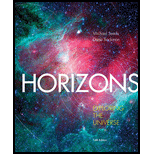Chapter 9, Problem 20RQHorizons: Exploring the Universe (...

14th Edition
Michael A. Seeds + 1 other
ISBN: 9781305960961

Solutions

Chapter
SectionHorizons: Exploring the Universe (...

14th Edition
Michael A. Seeds + 1 other
ISBN: 9781305960961
Textbook Problem

How Do We know? How can mathematical models help you understand natural processes that occur in locations or with time scales that make them impossible to observe directly?

To determine

How mathematical models help you understand natural process.

Explanation

A mathematical model is a description of a system using mathematical concepts and language. It is used to cast observed phenomenon into a mathematical description.

Mathematical models can make inferences about unobservable phenomena by looking at th...

Still sussing out bartleby?

Check out a sample textbook solution.

See a sample solution

The Solution to Your Study Problems

Bartleby provides explanations to thousands of textbook problems written by our experts, many with advanced degrees!

Get Started

Find more solutions based on key concepts